# 人智导（六）：“不可测”问题的求解

## 动作效果的不确定性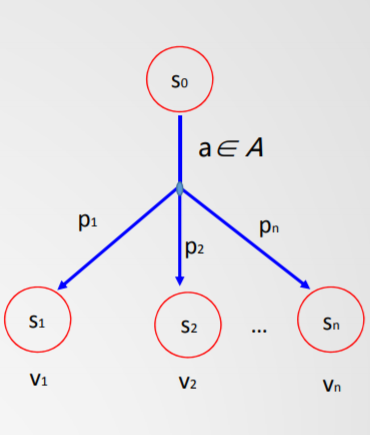• 智能体不能确切地直到其动作的效果，可能有多个结果状态
• 表示为：$[s_1,\dots ,s_n]=result(s_0,a)$
• 动作效果不确定，需要与环境交互
• 在执行动作之前，智能体需要计算所用结果状态的概率$P(s_i|a)$
• 动作的期望效用(Expected Utility)：$EU(a)=\Sigma_iP(s_i|a)V(s_i)$
• 状态价值函数$V(s)$：状态到实数值的映射
• 智能体应当选择当前状态下具有最大期望效用(MEU)的动作

## 最优策略

• 动作后续状态确定(deterministic)的搜索问题
• 发现最优(optimal)plan，从初始状态到目标状态的一个动作序列
• 动作后续状态不确定(nondeterministic)
• 发现一个最优(optimal)policy $\pi ^* :s\to a$
• policy策略为一个状态确定应采取的动作(what to do)
• 最优的policy是满足MEU的

## 不确定条件下的搜索问题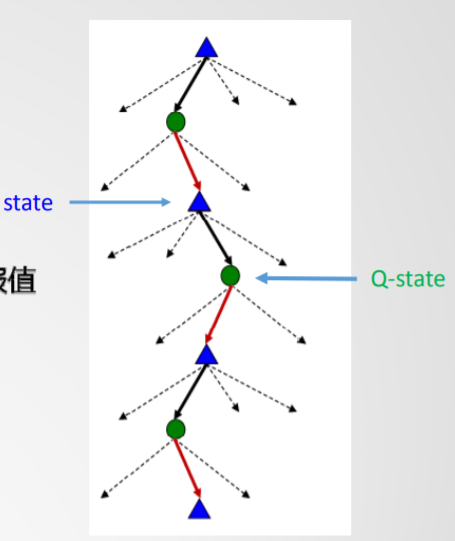• “不可测”问题
• 目标导向(goal-seeking)
• 与环境交互，只有动作执行后才能确定后续状态
• 趋向目标的累计回报(reward)，而非动作直接的回报值
• 求解方法
• 发现最优policy策略$\pi ^*: s\to a$
• 即在任何一个状态$s$，确定趋向目标的最佳动作$a$
• 定义Q-state状态表示（计算）EU

### 与环境交互模型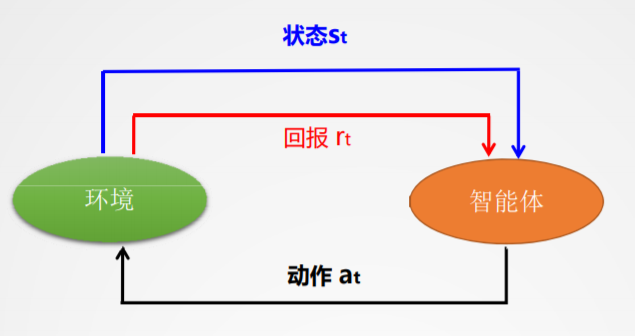### 马尔可夫决策过程

• 一个状态(state)集合：$S$
• 一个动作(actions)集合：$A$
• 一个后继函数$T(s,a,s')$，且从状态$s$$s'$的概率为$P(s'|s,a)$
• 一个回报(reward)函数：$R(s,a,s')$
• 初始状态$s_0$
• 一个或多个目标（结束）状态

## 马尔可夫过程

### 基本性质

$P(S_{t+1}=s'|S_t=s_t, A_t=a_t,S_{t-1}=s_{t-1},A_{t-1},\dots ,S_0=s_0)=P(S_{t+1}=s'|S_t=s_t,A_t=a_t)$

### Markov搜索树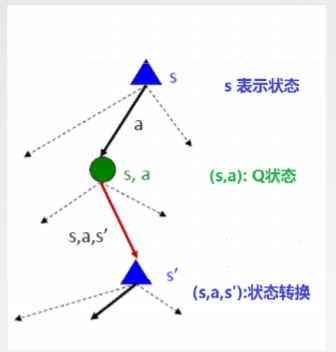MDP：求解动作效果不确定问题

• 对任意状态$s$下的动作选择：$policy~\pi^*(s):s\to a$
• 任意状态$s$选这样的动作，使得价值函数$V(s)$计算累计回报(sum of rewards)期望最大化

### 如何选择最优动作

• 对任意一个状态$s$，都通过价值函数对应一个值
• $V(s)=$累计回报最大期望值{目标状态$V$值为0}
• 最优策略：$\pi ^* =arg_{\pi}~max~V^{\pi}(s),(\forall s)$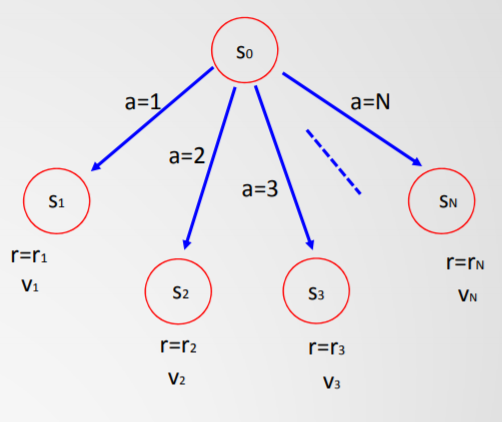$V_0=max_{a\in 1, \dots ,N}(r_a+\gamma V_a)$

• 非确定动作的最大期望值（如下图）$V_0=max_{a\in A}\Sigma _{s\in S}P_{a, s_0\to s}(r_s+\gamma V_s)$
• 同时体现了当前动作对后续进程的影响$V^{\pi}(s_t)=r_t +\gamma r_{t+1} +\gamma^2r_{t+2}+\dots =\Sigma^{\infty}_{i=0}\gamma ^i r_{t+i}$

### 引入$Q(s,a)$状态表示

• 状态$s$及其状态值：$V(s)=$始于$s$按最优策略行动的累计回报
• $Q(s,a)$的值：$Q(s,a)=EU(a)$，在$s$状态下执行$a$
• 最优策略policy:$\pi ^* (s)$ $\pi ^*=arg_{\pi}max~V^{|pi}(s),(\forall s)$

## 最优策略的计算

• 有如下等式$(0\le \gamma\le 1)$: $V(s)=max_a ~Q(s,a)$ $Q(s,a)=\Sigma_{s'}P(s,a,s')[r(s,a,s')+\gamma V(s')]$ $(Bellman~equation)~V(s)=max_a\Sigma_{s'}P(s,s,s')[r(s,a,s')+\gamma V(s')]$
• 迭代计算：$V_{k+1}(s) \leftarrow max_a\Sigma_{s'}P(s,a,s')[r(s,a,s')+\gamma V_k(s')]$
状态值迭代计算方法：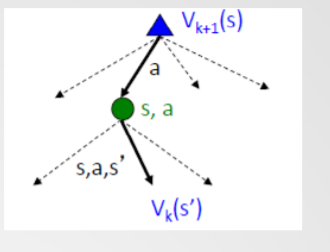状态空间：
$S=\{s_1, \dots ,s_n\}$
Bellman方程组（每个状态对应一个方程）：
$\left[ \begin{matrix} V_{s1}\\ \vdots \\ V_{sn} \end{matrix} \right] = \left[ \begin{matrix} P_{11} &\cdots &P_{1n}\\ \vdots &\ddots &\vdots \\ P_{n1} &\cdots &P_{nn} \end{matrix} \right] \left[ \begin{matrix} V_{s1}\\ \vdots \\ V_{sn} \end{matrix} \right]$
其中：
$P_{ij}=\begin{cases}p_{i\to j} &if~j\in successor(i) \\ 0 & otherwise \end{cases}$
向量表示：
$V_{k+1} = PV_k$
初始条件：
$V_0=\left[\begin{matrix}0\\ \vdots \\0 \end{matrix}\right]$

## 举例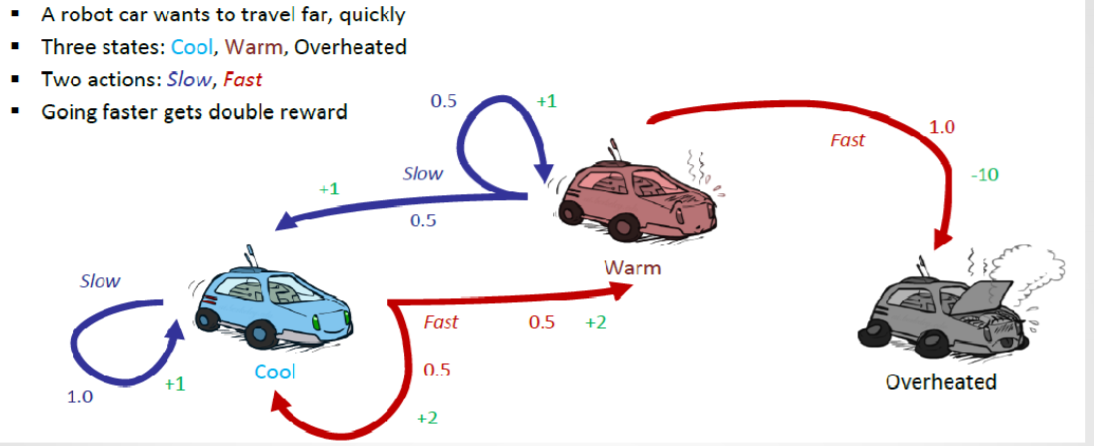MDP搜索树：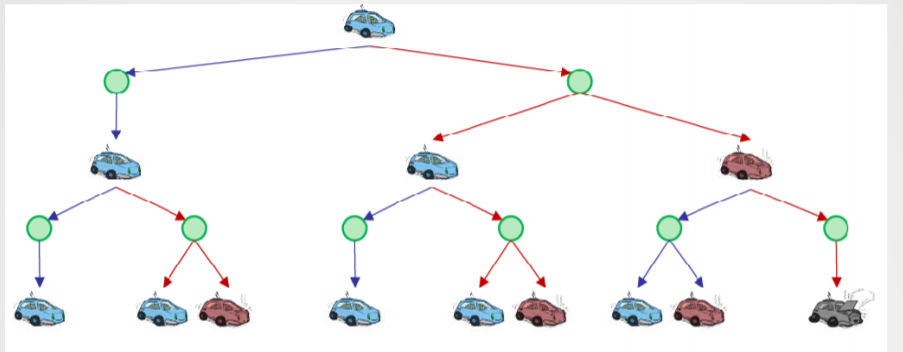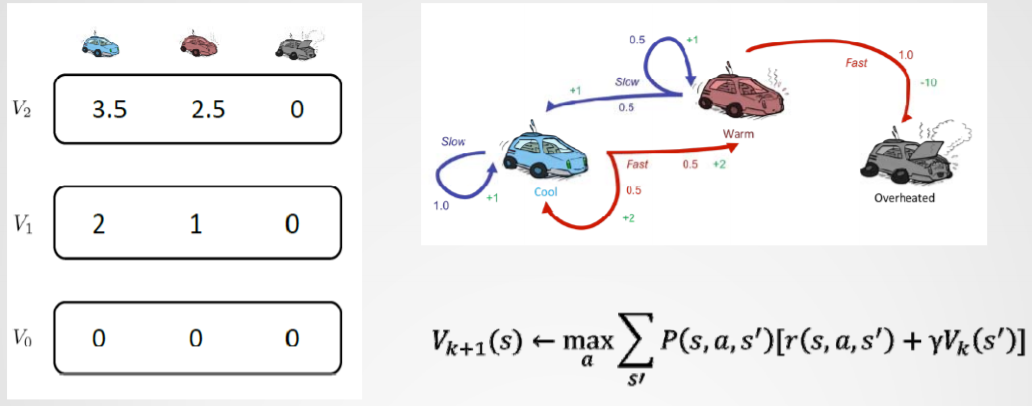05-24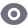3万+

#### 程序员编程艺术：第六章、求解500万以内的亲和数

11-025万+

#### 遗传算法 求解旅行商 TSP 问题，matlab代码

05-214269

#### 【Python学习笔记】13：用梯度下降法求解最优值问题

04-196231

#### C语言编程：用LU分解法求解方程组

06-257334

#### 人智导（四）：约束满足问题

03-145028

#### 致佳音: 推箱子游戏自动求解算法设计(一)

10-255097

#### 回溯法——求解N皇后问题

06-284287

#### 人智导（五）：对抗搜索与博弈

06-171万+

#### Python——使用scipy求解带约束的最优化问题

10-092万+

#### 基于粒子群算法求解TSP问题（JAVA）

10-174万+

#### 基于遗传算法求解TSP问题（JAVA）

10-285230

#### 回溯法——求解0-1背包问题

12-168597

#### ML之PLiR之LARS：利用LARS算法求解ElasticNet回归类型问题(实数值评分预测)

04-2537

#### 《第一行代码》读书笔记（五）：悬浮按钮爬坑

07-313917

#### 经典算法精讲精练之回溯法求解0-1背包问题

05-031万+

#### 基于蚁群算法求解求解TSP问题（JAVA）©️2020 CSDN 皮肤主题: 鲸 设计师: meimeiellie点击重新获取扫码支付1.余额是钱包充值的虚拟货币，按照1:1的比例进行支付金额的抵扣。
2.余额无法直接购买下载，可以购买VIP、C币套餐、付费专栏及课程。余额充值